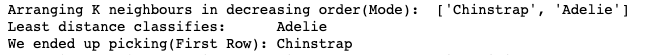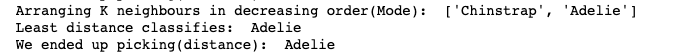# Exploring The Brute Force K-Nearest Neighbors Algorithm

By Murugan Yuvaraaj, Praxis Business SchooDid you find any difference between the two graphs?

Both show the accuracy of a classification problem for K values between 1 to 10.

Both of the graphs use the KNN classifier model with ‘Brute-force’ algorithm and ‘Euclidean’ distance metric on same dataset. Then why is there a difference in the accuracy between the two graphs?

Before answering that question, let me just walk you through the KNN algorithm pseudo code.

I hope all are familiar with k-nearest neighbour algorithm. If not, you can read the basics about it at https://www.analyticsvidhya.com/blog/2018/03/introduction-k-neighbours-algorithm-clustering/.

We can implement a KNN model by following the below steps:

2. Initialise the value of k
3. For getting the predicted class, iterate from 1 to total number of training data points
4. Calculate the distance between test data and each row of training data. Here we will use Euclidean distance as our distance metric since it’s the most popular method. Some of the other metrics that can be used are Chebyshev, cosine, etc.
5. Sort the calculated distances in ascending order based on distance values
6. Get top k rows from the sorted array
7. Get the most frequent class of these rows
8. Return the predicted class

For our analysis, lets just focus on step 7, getting the most frequent class of these rows.

After getting the top k rows, we pick the most frequent class (mode) from these rows. There is a little problem with that.

In case of an odd k neighbours, there will be always a majority class in the list. Thus, there will be no problem with odd k neighbours.

But what about an even k neighbours number and if two or more classes get the same majority?

The KNN algorithm can also give high accuracy for a dataset for k even neighbours. It is not restricted to only use odd k neighbours to get the majority class.

Take for example:

If k = 4 and we have Class A = 2 and Class B = 2 in our list. In that case, the algorithm will take the class what falls in the first rows of the top K rows instead of looking at the distance metric.

To solve this problem, we used distance – mode – distance as our criteria for even-numbered k neighbours in our algorithm.

Our algorithm works the same way as the brute-force algorithm, but the difference that it makes with even k neighbours is great.

What our algorithm does is very simple. It takes the top k rows from the distance metric. In the case of an odd k value, it takes the majority. For an even number of k rows, majority classes are selected. If it happens to have two or more classes having a majority, those two or more major class distances will go to the distance metric loop again and check which class has the lowest distance metric, and that class is chosen as the majority class.

Let’s see an example of how this works.

For our analysis we used the penguin dataset.

Brute-force Algorithm:Here we gave k = 4.

Class ‘Chinstrap’ and ‘Adelie’ ended up with mode as 2. After arranging the K neighbours based on mode, brute-force ended up picking the first class instead of picking the class which had least distance in the distance metric.

This affects the accuracy for the brute-force algorithm when k value is even.

Our Model:Our model is able to increase the accuracy in case of even numbered neighbours.

Results:

I have compared the accuracy of our model with brute-force and below are the results.

 K Brute Force Our Model 1 0.805 0.805 2 0.746 0.805 3 0.761 0.761 4 0.746 0.791 5 0.776 0.776 6 0.716 0.791 7 0.746 0.746 8 0.686 0.746 9 0.746 0.746 10 0.701 0.776

I compared the results with kd tree and ball tree algorithms also, and similar results were obtained.

Thanks for your time and be kind with your feedback as this is my first article.

Bio: Murugan Yuvaraaj is a Student at Praxis Business School in Bangalore, India.

Related: